Today's lab will use decisions to extend our simple Tic-Tac-Toe game to print who has won the game. In the second part of the lab, we will also revisit finding errors.

Turtle Tic-Tac-Toe (Version 1)

For today's lab, we're going to extend a very simple Tic-Tac-Toe program from Lab 4.

Our first program allowed the user to specify moves. Below is the program organized into functions:

#Introductory Program, Spring 2015
#Lehman College, City University of New York
#First Version of Tic-Tac-Toe
#   This version does NO checking of anything (it doesn't
#   check who wins, doesn't check for legal entries, etc).
#   We will add that later in the semester

from turtle import *

def setUp():
#Set up the screen and turtle
win = Screen()
tic = Turtle()
tic.speed(10)
#Change the coordinates to make it easier to tranlate moves to screen coordinates:
win.setworldcoordinates(-0.5,-0.5,3.5, 3.5)

#Draw the vertical bars of the game board:
for i in range(1,3):
tic.up()
tic.goto(0,i)
tic.down()
tic.forward(3)

#Draw the horizontal bars of the game board:
tic.left(90)    #Point the turtle in the right direction before drawing
for i in range(1,3):
tic.up()
tic.goto(i,0)
tic.down()
tic.forward(3)

tic.up()        #Don't need to draw any more lines, so, keep pen up
return(win,tic)

def playGame(tic):
#Ask the user for the first 8 moves, alternating between the players X and O:
for i in range(4):
x,y = eval(input("Enter x, y coordinates for X's move: "))
tic.goto(x+.25,y+.25)
tic.write("X",font=('Arial', 90, 'normal'))
x,y = eval(input("Enter x, y coordinates for O's move: "))
tic.goto(x+.25,y+.25)
tic.write("O",font=('Arial', 90, 'normal'))

# The ninth move:
x,y = eval(input("Enter x, y coordinates for X's move: "))
tic.goto(x+.25,y+.25)
tic.write("X",font=('Arial', 90, 'normal'))

def cleanUp(tic,win):
#Display an ending message:
tic.goto(-0.25,-0.25)
tic.write("Thank you for playing!",font=('Arial', 20, 'normal'))

win.exitonclick()#Closes the graphics window when mouse is clicked

def main()
win,tic = setUp()   #Set up the window and game board
playGame(tic)       #Ask the user for the moves and display
#print("\nThe winner is", checkWinner(board))  #Check for winner
cleanUp(tic,win)    #Display end message and close window

main()
We would like to print if there is a winner for our game (the line in the main that is commented out). Our current program asks the user for the move, draws it to the graphics windown, but does not save the move. If we are going to check for winners, we will need to save the moves. We can do this using a lists:
board = [["","",""],["","",""],["","",""]]
Each position corresponds to a position on the screen and all are currently set to empty strings. But, each time someone makes a move, we can add in the move to the list board so that we can check at the end who has one. Every time you draw a move on the graphics window, also save it in the game board. For example, if user "X" chooses (x,y), then we can store that move by:
board[x][y] = "X"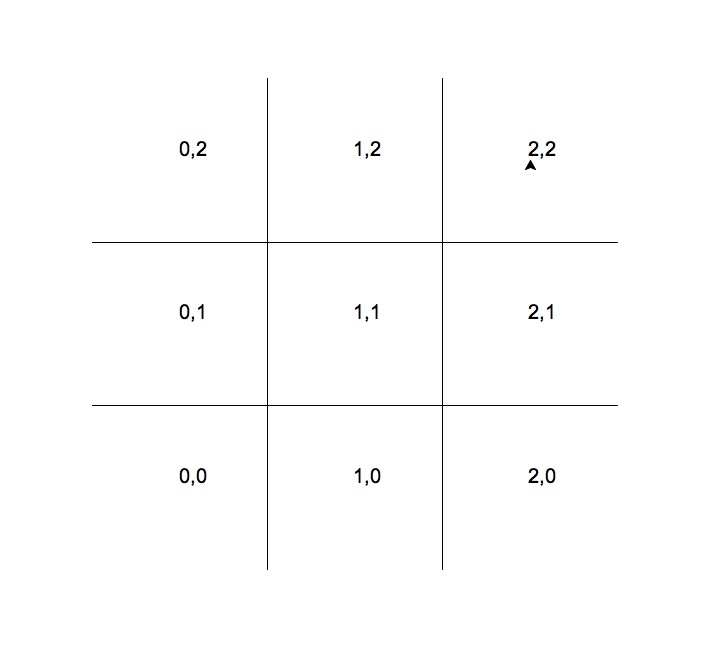Modify the end of setUp() include setting up the game board and returning it:

#Set up board:
board = [["","",""],["","",""],["","",""]]

return(win,tic,board)
Modify the playGame() function to stores moves:
def playGame(tic,board):
#Ask the user for the first 8 moves, alternating between the players X and O:
for i in range(4):
x,y = eval(input("Enter x, y coordinates for X's move: "))
tic.goto(x+.25,y+.25)
tic.write("X",font=('Arial', 90, 'normal'))
board[x][y] = "X"

x,y = eval(input("Enter x, y coordinates for O's move: "))
tic.goto(x+.25,y+.25)
tic.write("O",font=('Arial', 90, 'normal'))
board[x][y] = "O"

# The ninth move:
x,y = eval(input("Enter x, y coordinates for X's move: "))
tic.goto(x+.25,y+.25)
tic.write("X",font=('Arial', 90, 'normal'))
board[x][y] = "X"
def checkWinner(board):
return("No winner")
(we will add more to this).

Lastly, change your main() function to keep track of board moves:

def main():
win,tic,board = setUp()   #Set up the window and game board
playGame(tic,board)       #Ask the user for the moves and display
print("The winner is", checkWinner(board))  #Check for winner
cleanUp(tic,win)    #Display end message and close window
Try your program. What happens at the end? Who is the winner? Is it always the same? Why?

Our current program does no checking but just returns "No Winner" no matter what is played. We will add in checks to see who has won. Let's start with the row (0,0), (1,0), and (2,0). What does it mean for someone to win on that row? The entries have to be non-empty and all the same:

def checkWinner(board):
if board != "" and (board== board == board):
return(board)  #we have a non-empty row that's identical
return("No winner")
Note that if the row is identical and non-empty, then the conditional test is true, and the return command is performed, leaving the function immediately.

Since the same pattern holds for the rows where x=1 and x=2, we can put the if-statement into a loop:

def checkWinner(board):
for x in range(3):
if board[x] != "" and (board[x]== board[x] == board[x]):
return(board[x])  #we have a non-empty row that's identical
return("No winner")
Try adding in the same check for the columns without peeking below. Here's the answer if you get stuck:
def checkWinner(board):
for x in range(3):
if board[x] != "" and (board[x]== board[x] == board[x]):
return(board[x])  #we have a non-empty row that's identical
for y in range(3):
if board[y] != "" and (board[y]== board[y] == board[y]):
return(board[y])  #we have a non-empty row that's identical
return("No winner")
Lastly, we need to check for winners on the diagonal. That is, the moves at (0,0), (1,1), and (2,2) are identical (and non-empty) or (0,2), (1,1), and (2,0) are identical (and non-empty). Try writing this before looking at the answer below:
def checkWinner(board):
for x in range(3):
if board[x] != "" and (board[x] == board[x] == board[x]):
return(board[x])  #we have a non-empty row that's identical
for y in range(3):
if board[y] != "" and (board[y] == board[y] == board[y]):
return(board[y])  #we have a non-empty column that's identical
if board != "" and (board == board == board):
return(board)
if board != "" and (board == board == board):
return(board)
return("No winner")
Try playing the game several times. What happens if there is no winner? What happens if there is more than one? What happens if both players chose the same move? Whose move is counted when computing the winner? Why?

The complete program:

#Introductory Program, Spring 2015
#Lehman College, City University of New York
#Second Version of Tic-Tac-Toe
#   This version checks the number of winning
#	positions at the end of the game.

from turtle import *

def setUp():
#Set up the screen and turtle
win = Screen()
tic = Turtle()
tic.speed(10)
#Change the coordinates to make it easier to tranlate moves to screen coordinates:
win.setworldcoordinates(-0.5,-0.5,3.5, 3.5)

#Draw the vertical bars of the game board:
for i in range(1,3):
tic.up()
tic.goto(0,i)
tic.down()
tic.forward(3)

#Draw the horizontal bars of the game board:
tic.left(90)    #Point the turtle in the right direction before drawing
for i in range(1,3):
tic.up()
tic.goto(i,0)
tic.down()
tic.forward(3)

tic.up()        #Don't need to draw any more lines, so, keep pen up

#Set up board:
board = [["","",""],["","",""],["","",""]]

return(win,tic,board)

def playGame(tic,board):
#Ask the user for the first 8 moves, alternating between the players X and O:
for i in range(4):
x,y = eval(input("Enter x, y coordinates for X's move: "))
tic.goto(x+.25,y+.25)
tic.write("X",font=('Arial', 90, 'normal'))
board[x][y] = "X"

x,y = eval(input("Enter x, y coordinates for O's move: "))
tic.goto(x+.25,y+.25)
tic.write("O",font=('Arial', 90, 'normal'))
board[x][y] = "O"

# The ninth move:
x,y = eval(input("Enter x, y coordinates for X's move: "))
tic.goto(x+.25,y+.25)
tic.write("X",font=('Arial', 90, 'normal'))
board[x][y] = "X"

def checkWinner(board):
for x in range(3):
if board[x] != "" and (board[x] == board[x] == board[x]):
return(board[x])  #we have a non-empty row that's identical
for y in range(3):
if board[y] != "" and (board[y] == board[y] == board[y]):
return(board[y])  #we have a non-empty column that's identical
if board != "" and (board == board == board):
return(board)
if board != "" and (board == board == board):
return(board)
return("No winner")

def cleanUp(tic,win):
#Display an ending message:
tic.goto(-0.25,-0.25)
tic.write("Thank you for playing!",font=('Arial', 20, 'normal'))

win.exitonclick()#Closes the graphics window when mouse is clicked

def main():
win,tic,board = setUp()   #Set up the window and game board
playGame(tic,board)       #Ask the user for the moves and display
print("\nThe winner is", checkWinner(board))  #Check for winner
cleanUp(tic,win)    #Display end message and close window

main()

Finding Errors, II

In Lab 7, we looked at some common syntax errors and how to fix them. Most of those errors were missing punctuation (such at colons, quotes, or plus signs). Let's look at a few more errors that occur when using conditionals, loops, and functions.

Load the following file into IDLE, and then try to solve as many of these errors on your own. If you get stuck, refer to images and explanations below:

# errors2.py-- modified from Zelle
# recursions.py
#   A collection of simple recursive functions from Zelle, 2nd Edition
#   (Some also include looping counterparts).

def fact(n)
# returns factorial of n
if n == 0:
return 1
else
return n * fact(n-1

def reverse(s):
# returns reverse of string s
if s == "":
return s
elif:
return reverse(s[1:]) + s

def anagrams(s):
# returns a list of all anagrams of string s
if s == "":
return [s]
else:
ans = []
for w in anagrams(s[1:]):
for pos in range(len(w)+1):
ans.append(w[:pos]+s+w[pos:])
return ans

def loopFib(n):
# returns the nth Fibonacci number
curr = 1
prev = 1
for i in range(n-2):
curr, prev = curr+prev, curr
return curr

def main():
n = eval(input("Enter a number: "))
s = input("Enter a string: ")
print(n+"!= ", fact(n), "or, loopFig(n))
print(s, "reversed is: ", reverse(s))
print("\n anagrams: ", anagrams(s))

main()

Load the program into IDLE and run the program. A dialog box pops up and says "invalid syntax":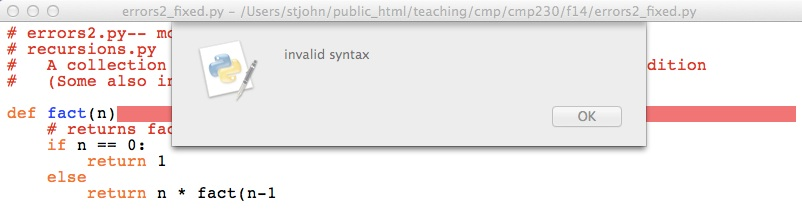We have seen this one before, it's a missing colon (":") at the end of the function definition. Add it in and run again.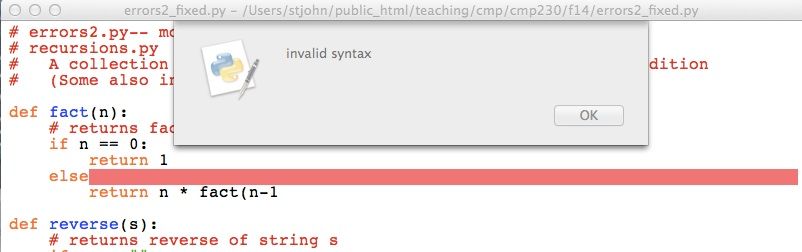Again, we get an invalid syntax. What's wrong here? (Hint: same as the last one). Fix the error, and let's run the program again: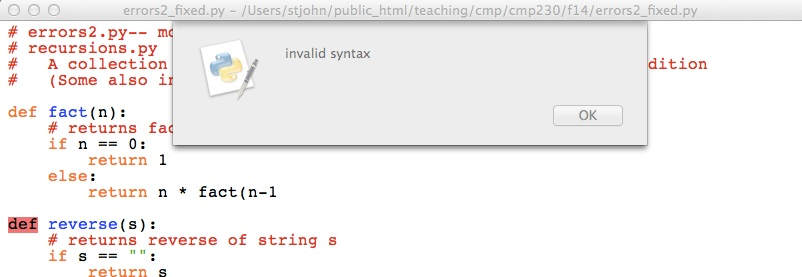This one is different. IDLE has highlighted the word def, and yet that seems to spelled correctly. A general rule to follow: if you do not see an error on the current line, look above (usually for missing quotes or closing parenthesis). On the previous line, the function call to fact() is missing the closing parenthesis. Add it, and run the program again: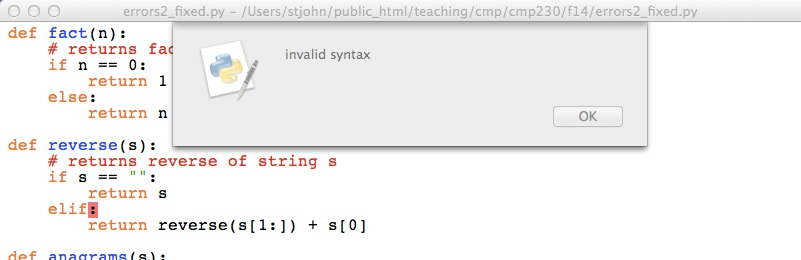This one is a bit harder-- all the keywords are spelled correctly and there's no missing colons, parenthesis, or quotes. What's wrong? Python expected the word else (elif is only used when you need multiple tests in multi-way decision). Replace elif with else and try again: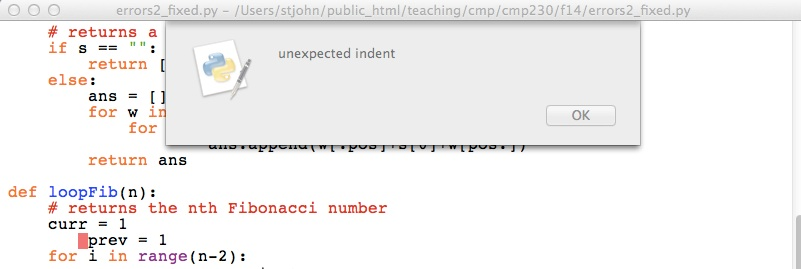The message says: unexpected indent. The line does seem to be indented for no reason (i.e. it's not part of a block of code in a loop or decision construct). Remove the extra indent and continue: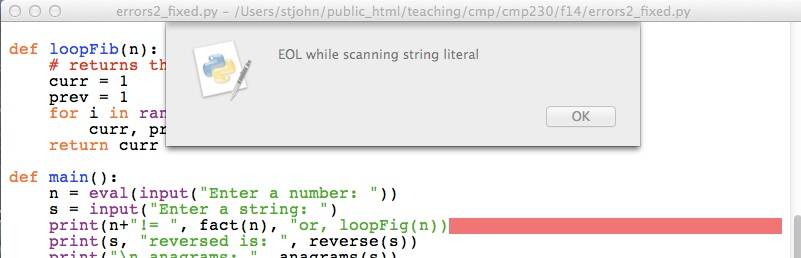This is a common error. In plain English, it means that the end of the line ("EOL") was reached before the string finished. In other words, the string is missing its ending quotes. Add it in (right after the word or) and run the program again.

It now compiles! But we need to test it to make sure no run-time errors remain: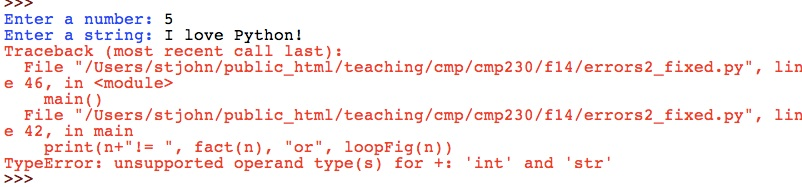When reading the traceback message (all the red text), go to the very last line and see what it says. The message says we cannot use + for 'int' and 'string'. We only used + once in the line to concatenate n to a string. Since we want n to eventually be a string and printed to the screen, let's change it to a string (by using the str() function):

print(str(n)+"!= ", fact(n), "or", loopFig(n))

Running it again, a new run-time errors appears: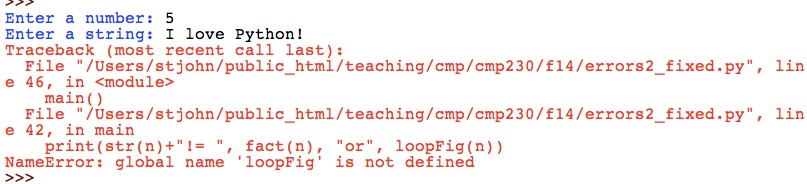The message says that IDLE cannot find loopFig(). Scanning through the file, it looks we misspelled it! The function is called loopFib(). Fix the misspelling in the main() and try again...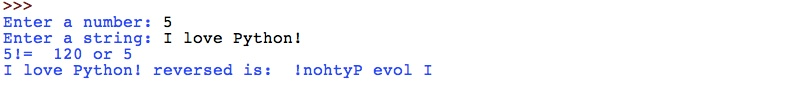Success! The program accepted input, processed it, and outputted it to the screen. Try with a couple of different inputs to test how it works.

In-class Quiz

During lab, there is a quiz on functions and loops. The password to access the quiz will be given during lab.

What's Next?

If you finish the lab early, now is a great time to get a head start on the programming problems due early next week. There's instructors to help you and you already have Python up and running. The Programming Problem List has problem descriptions, suggested reading, and due dates next to each problem.